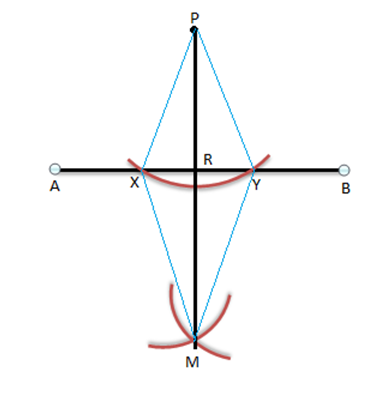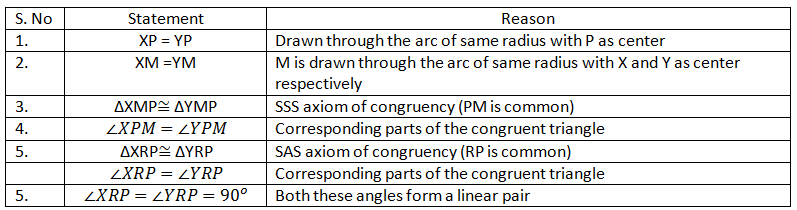# Construction of Perpendicular Line Through a PointLines which intersect each other at right angles are perpendicular to each other. In other words, if two lines are perpendicular to each other then, the angle made by them at the point of intersection will be 90o and vice versa. In the upcoming discussion, we shall discuss how to construct a  perpendicular line to another line through an external point.

## Constructing a Perpendicular to a Line through an External Point

Given: A line segment AB and a point P lying outside the line segment AB

To construct: A line perpendicular to line AB passing through point P

### Steps for Construction

• Step 1:With point P as center and any suitable radius draw an arc cutting line segment AB at two distinct points X and Y as shown in the following figure.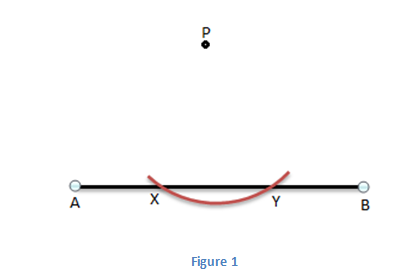• Step 2: Now with X as center and a suitable radius draw an arc on opposite side of the given point P. Also, with Y as center and same radius as previous, draw an arc on opposite side of the given point P cutting the arc drawn through point X at M as shown in the following figure.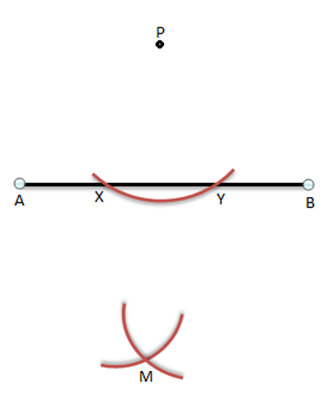• Step 3: Join the points P and M. The line segment PM is the required perpendicular to the line segment AB and passing through external point P.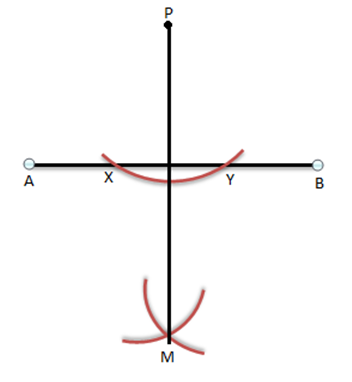To check if the line segment constructed in the way as discussed is perpendicular to line segment AB, join the points M and P to X and Y as shown below: